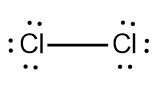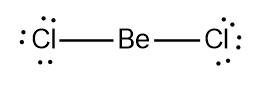# Problem: Calculate the formal charge of chlorine in the molecules Cl2, BeCl2, and ClF5.

###### FREE Expert Solution

We are asked to calculate the formal charge of chlorine in the molecules Cl2, BeCl2, and ClF5.

The ideal configuration of Cl is to have 1 bond and 3 lone pairs.

Cl2 Lewis structure:

Cl → Group 7A → 7 ve

Total = 14 veThe formal charge of Cl in Cl2 is 0.

BeCl2 Lewis structure:

Cl → Group 7A → 2 x 7 ve = 14 ve

Be → Group 2A → 2 ve

Total = 16 veThe formal charge of Cl in BeCl2 is 0.

88% (170 ratings)###### Problem Details

Calculate the formal charge of chlorine in the molecules Cl2, BeCl2, and ClF5.

Frequently Asked Questions

What scientific concept do you need to know in order to solve this problem?

Our tutors have indicated that to solve this problem you will need to apply the Lewis Dot Structure: Formal Charge concept. If you need more Lewis Dot Structure: Formal Charge practice, you can also practice Lewis Dot Structure: Formal Charge practice problems.

What professor is this problem relevant for?

Based on our data, we think this problem is relevant for Professor Cook's class at American Public University System.

What textbook is this problem found in?

Our data indicates that this problem or a close variation was asked in Chemistry - OpenStax 2015th Edition. You can also practice Chemistry - OpenStax 2015th Edition practice problems.